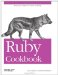# Finding Mean, Median, and Mode

Problem

You want to find the average of an array of numbers: its mean, median, or mode.

Solution

Usually when people speak of the "average" of a set of numbers they're referring to its mean, or arithmetic mean. The mean is the sum of the elements divided by the number of elements.

```	def mean(array)
array.inject(array.inject(0) { |sum, x| sum += x } / array.size.to_f
end

mean([1,2,3,4]) # => 2.5
mean([100,100,100,100.1]) # => 100.025
mean([-100, 100]) # => 0.0
mean([3,3,3,3]) # => 3.00
```

The median is the item x such that half the items in the array are greater than x and the other half are less than x. Consider a sorted array: if it contains an odd number of elements, the median is the one in the middle. If the array contains an even number of elements, the median is defined as the mean of the two middle elements.

```	def median(array, already_sorted=false)
return nil if array.empty?
m_pos = array.size / 2
return array.size % 2 == 1 ? array[m_pos] : mean(array[m_pos-1..m_pos])
end

median([1,2,3,4,5]) # => 3
median([5,3,2,1,4]) # => 3
median([1,2,3,4]) # => 2.5
median([1,1,2,3,4]) # => 2
median([2,3,-100,100]) # => 2.5
median([1, 1, 10, 100, 1000]) # => 10
```

The mode is the single most popular item in the array. If a list contains no repeated items, it is not considered to have a mode. If an array contains multiple items at the maximum frequency, it is "multimodal." Depending on your application, you might handle each mode separately, or you might just pick one arbitrarily.

```	def modes(array, find_all=true)
histogram = array.inject(Hash.new(0)) { |h, n| h[n] += 1; h }
modes = nil
histogram.each_pair do |item, times|
modes << item if modes && times == modes and find_all
modes = [times, item] if (!modes && times>1) or (modes && times>modes)
end
return modes ? modes[1…modes.size] : modes
end

modes([1,2,3,4]) # => nil
modes([1,1,2,3,4]) # => 
modes([1,1,2,2,3,4]) # => [1, 2]
modes([1,1,2,2,3,4,4]) # => [1, 2, 4]
modes([1,1,2,2,3,4,4], false) # => 
modes([1,1,2,2,3,4,4,4,4,4]) # => 
```

Discussion

The mean is the most popular type of average. It's simple to calculate and to understand. The implementation of mean given above always returns a floating-point number object. It's a good general-purpose implementation because it lets you pass in an array of Fixnums and get a fractional average, instead of one rounded to the nearest integer. If you want to find the mean of an array of BigDecimal or Rational objects, you should use an implementation of mean that omits the final to_f call:

```	def mean_without_float_conversion(array)
array.inject(0) { |x, sum| sum += x } / array.size
end
require 'rational'
numbers = [Rational(2,3), Rational(3,4), Rational(6,7)]
mean(numbers)
# => 0.757936507936508
mean_without_float_conversion(numbers)
# => Rational(191, 252)
```

The median is mainly useful when a small proportion of outliers in the dataset would make the mean misleading. For instance, government statistics usually show "median household income" instead of "mean household income." Otherwise, a few super-wealthy households would make everyone else look much richer than they are. The example below demonstrates how the mean can be skewed by a few very high or very low outliers.

```	mean([1, 100, 100000]) # => 33367.0
median([1, 100, 100000]) # => 100

mean([1, 100, -1000000]) # => -333299.666666667
median([1, 100, -1000000]) # => 1
```

The mode is the only definition of "average" that can be applied to arrays of arbitrary objects. Since the mean is calculated using arithmetic, an array can only be said to have a mean if all of its members are numeric. The median involves only comparisons, except when the array contains an even number of elements: then, calculating the median requires that you calculate the mean.

If you defined some other way to take the median of an array with an even number of elements, you could take the median of Arrays of strings:

```	median(["a", "z", "b", "l", "m", "j", "b"])
# => "j"
median(["a", "b", "c", "d"])
# TypeError: String can't be coerced into Fixnum
```

The standard deviation

A concept related to the mean is the standard deviation, a quantity that measures how close the dataset as a whole is to the mean. When a mean is distorted by high or low outliers, the corresponding standard deviation is high. When the numbers in a dataset cluster closely around the mean, the standard deviation is low. You won't be fooled by a misleading mean if you also look at the standard deviation.

```	def mean_and_standard_deviation(array)
m = mean(array)
variance = array.inject(0) { |variance, x| variance += (x - m) ** 2 }
return m, Math.sqrt(variance/(array.size-1))
end

#All the items in the list are close to the mean, so the standard
#deviation is low.
mean_and_standard_deviation([1,2,3,1,1,2,1])
# => [1.57142857142857, 0.786795792469443]
#The outlier increases the mean, but also increases the standard deviation.
mean_and_standard_deviation([1,2,3,1,1,2,1000])
# => [144.285714285714, 377.33526837801]
```

A good rule of thumb is that two-thirds (about 68 percent) of the items in a dataset are within one standard deviation of the mean, and almost all (about 95 percent) of the items are within two standard deviations of the mean.

• "Programmers Need to Learn Statistics or I Will Kill Them All," by Zed Shaw (http://www.zedshaw.com/blog/programming/programmer_stats.html)
• More Ruby implementations of simple statistical measures (http://dada.perl.it/shootout/moments.ruby.html)
• To do more complex statistical analysis in Ruby, try the Ruby bindings to the GNU Scientific Library (http://ruby-gsl.sourceforge.net/)
• The Stats class in the Mongrel web server (http://mongrel.rubyforge.org) implements other algorithms for calculating mean and standard deviation, which are faster if you need to repeatedly calculate the mean of a growing seriesRuby Cookbook (Cookbooks (OReilly))
ISBN: 0596523696
EAN: 2147483647
Year: N/A
Pages: 399